Next: The Environment of the Up: ``Ecology'' of Magnetic Rotators Previous: ``Ecology'' of Magnetic Rotators

## A Gravimagnetic Rotator

ĀĀ

In this review, we shall call any gravitationally-bounded object having an angular momentum and intrinsic magnetic fieldĀ by the term ``gravimagnetic rotator''Ā or simply, rotator. In order to specify the intrinsic properties of the rotator, three parameters are sufficient - the mass M, the total angular momentum(I is the moment of inertia andis the angular velocity), and the magnetic dipole moment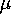(see FigureĀ3). Given the rotator radius, one can express the magnetic fieldĀ strength at the poles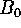by using the dipole moment. The angle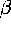between the angular momentand the magnetic dipole moment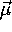can also be of importance: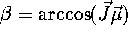.

Ā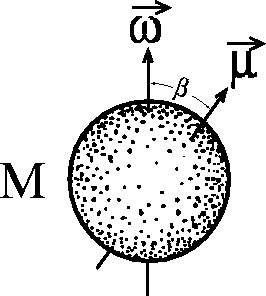Figure 3: Schematic representation of a gravimagnetic rotator.Ā

Mike E. Prokhorov
Sat Feb 22 18:38:13 MSK 1997